0
2013
Impact Factor

# On Nonlinear Resonant Oscillations of a Rigid Body Generated by Its Conical Precession

2018, Vol. 14, no. 4, pp.  503-518

Author(s): Markeev A. P.

The motion of a dynamically symmetric rigid body relative to its center of mass in the central Newtonian gravitational field in a circular orbit is investigated. This problem involves motion (called conical precession) where the dynamical symmetry axis of the body is located all the time in the plane perpendicular to the velocity vector of the center of mass of the body and makes a constant angle with the direction of the radius vector of the center of mass relative to the attracting center. This paper deals with a special case in which this angle is $\pi/4$ and the ratio between the polar and the equatorial principal central moments of inertia of the body is equal to the number $2/3$ or is close to it. In this case, the conical precession is stable with respect to the angles that define the position of the symmetry axis in an orbital coordinate system and with respect to the time derivatives of these angles, and the frequencies of small (linear) oscillations of the symmetry axis are equal or close to each other (that is, the 1:1 resonance takes place). Using classical perturbation theory and modern numerical and analytical methods of nonlinear dynamics, a solution is presented to the problem of the existence, bifurcations and stability of periodic motions of the symmetry axis of a body which are generated from its relative (in the orbital coordinate system) equilibrium corresponding to conical precession. The problem of the existence of conditionally periodic motions is also considered.
Keywords: resonance, stability, oscillations, canonical transformations
Citation: Markeev A. P., On Nonlinear Resonant Oscillations of a Rigid Body Generated by Its Conical Precession, Rus. J. Nonlin. Dyn., 2018, Vol. 14, no. 4, pp.  503-518
DOI:10.20537/nd180406

## References

 Beletskii, V. V., Motion of an Artificial Satellite about Its Center of Mass, Jerusalem, 1966, x, 261 pp.
 Beletskii, V. V., Satellite's Motion about Center of Mass in a Gravitational Field, MGU, Moscow, 1975 (Russian)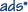Markeev, A. P., Linear Hamiltonian Systems and Some Problems of Stability of the Satellite Center of Mass, R&C Dynamics, Institute of Computer Science, Izhevsk, 2009, 396 pp. (Russian)
 Korteweg, D. J., “Sur certaines vibrations d'ordre supérieur et d'intensité anormale — vibrations de relations, — dans les mécanismes à plusieurs degrés de liberté”, Arch. Néerl. sci. exactes et natur. Sér. 2, 1 (1898), 229–260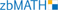Beth, H. I. E., “Les oscillations autour d'une position dans le cas d'existence d'une relation linéaire simple entre les nombres vibratoires”, Arch. Néerl. sci. exactes et natur. Sér. 2, 15 (1910), 246–283Beth, H. I. E., “Les oscillations autour d'une position dans le cas d'existence d'une relation linéaire simple entre les nombres vibratoires (suite)”, Arch. Néerl. sci. exactes et natur. Sér. 3A, 1 (1912), 185–213Markeyev, A. P., “Non-Linear Oscillations of a $1:1$ Resonance Hamiltonian System”, J. Appl. Math. Mech., 75:6 (2011), 631–646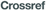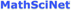; Prikl. Mat. Mekh., 75:6 (2011), 901–922 (Russian)Hénon, M. and Heiles, C., “The Applicability of the Third Integral of Motion: Some Numerical Experiments”, Astronom. J., 69:1 (1964), 73–79Roels, J., “An Extension to Resonant Cases of Liapunov's Theorem Concerning the Periodic Solutions near a Hamiltonian Equilibrium”, J. Differential Equations, 9:2 (1971), 300–324Braun, M., “On the Applicability of the Third Integral of Motion”, J. Differential Equations, 13:2 (1973), 300–318Breiter, S. and Elipe, A., “Pseudo-Oscillator with a Quartic Perturbation”, Mech. Res. Comm., 28:2 (2001), 119–126Kholostova, O. V. and Safonov, A. I., “Investigation of the Motions of an Autonomous Hamiltonian System at a $1:1$ Resonance”, Regul. Chaotic Dyn., 22:7 (2017), 792–807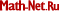Birkhoff, G. D., Dynamical Systems, AMS, Providence, R.I., 1966, 305 pp.Giacaglia, G. E. O., Perturbation Methods in Non-Linear Systems, Appl. Math. Sci., 8, Springer, New York, 1972, viii+369 pp.Markeyev, A. P., “The Critical Case of a Pair of Zero Roots in a Two-Degree-of-Freedom Hamiltonian System”, J. Appl. Math. Mech., 62:3 (1998), 341–349; Prikl. Mat. Mekh., 62:3 (1998), 372–382 (Russian)Markeev, A. P., “On Stability and Non-Linear Oscillations of Hamiltonian System in a Resonant Case”, Mech. Solids, 33:4 (1998), 32–41; Izv. Ross. Akad. Nauk. Mekh. Tverd. Tela, 1998, no. 4, 38–49 (Russian)
 Arnol'd, V. I., Kozlov, V. V., and Neĭshtadt, A. I., Mathematical Aspects of Classical and Celestial Mechanics, Encyclopaedia Math. Sci., 3, 3rd ed., Springer, Berlin, 2006, xiv+518 pp.Markeev, A. P., “Nonlinear Oscillations of Sympathetic Pendulums”, Nelin. Dinam., 6:3 (2010), 605–621 (Russian)Markeyev, A. P., “An Algorithm for Normalizing Hamiltonian Systems in the Problem of the Orbital Stability of Periodic Motions”, J. Appl. Math. Mech., 66:6 (2002), 889–896; Prikl. Mat. Mekh., 66:6 (2002), 929–938 (Russian)Neishtadt, A. I., “Estimates in the Kolmogorov Theorem on Conservation of Conditionally Periodic Motions”, J. Appl. Math. Mech., 45:6 (1981), 766–772; Prikl. Mat. Mekh., 45:6 (1981), 1016–1025 (Russian)